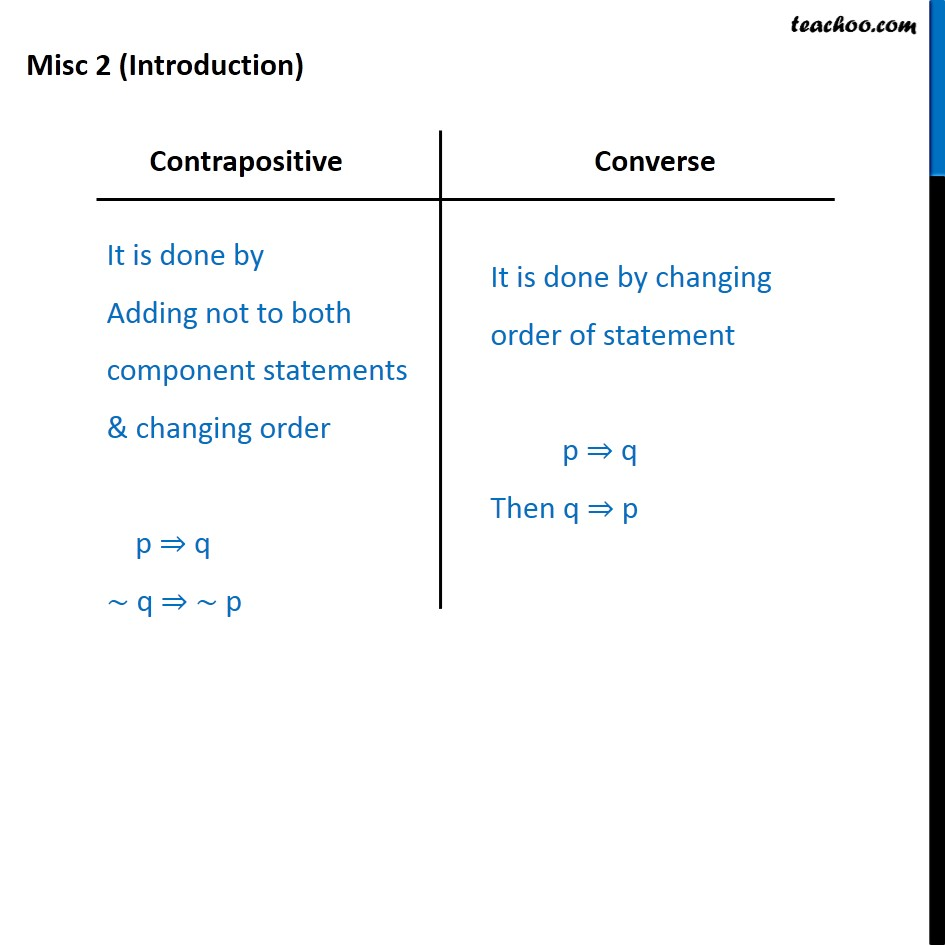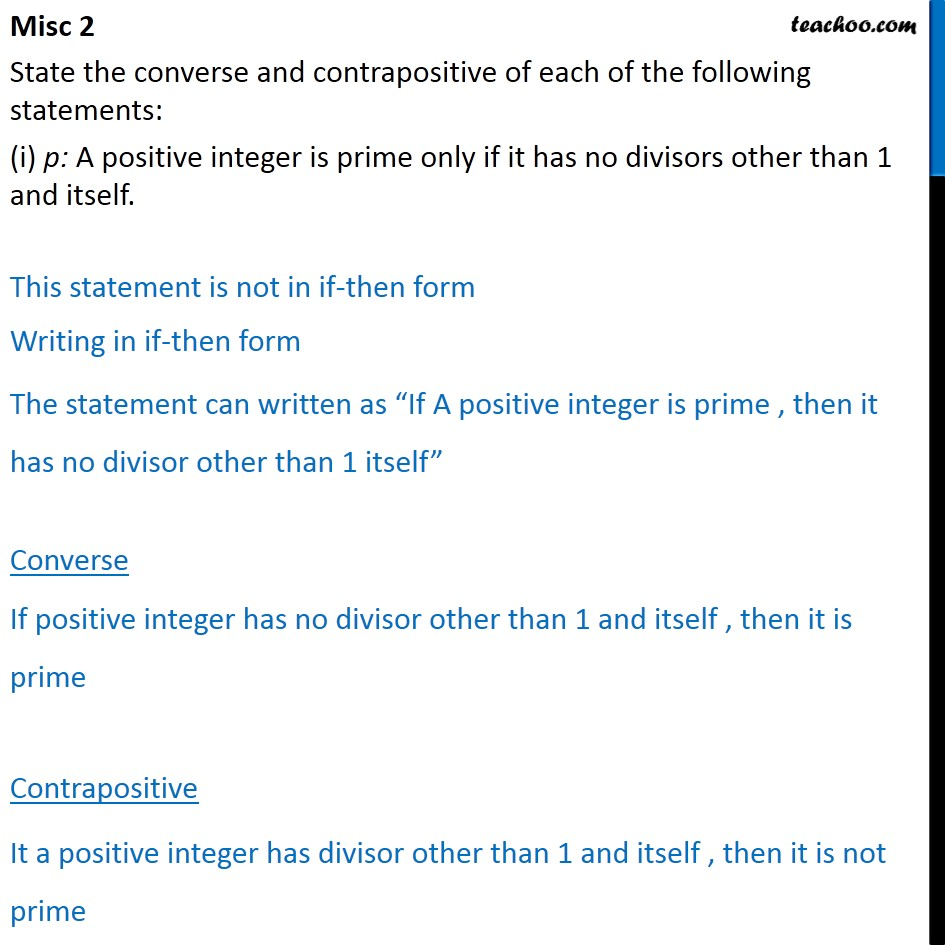Miscellaneous

Chapter 14 Class 11 Mathematical Reasoning (Deleted)
Serial order wise983 students joined Teachoo Black. What are you waiting for?

### Transcript

Misc 2 (Introduction) Contrapositive It is done by Adding not to both component statements & changing order p q ~ q ~ p Converse It is done by changing order of statement p q Then q p State the converse and contrapositive of each of the following statements: (i) p: A positive integer is prime only if it has no divisors other than 1 and itself. This statement is not in if-then form Writing in if-then form The statement can written as If A positive integer is prime , then it has no divisor other than 1 itself Converse If positive integer has no divisor other than 1 and itself , then it is prime Contrapositive It a positive integer has divisor other than 1 and itself , then it is not prime